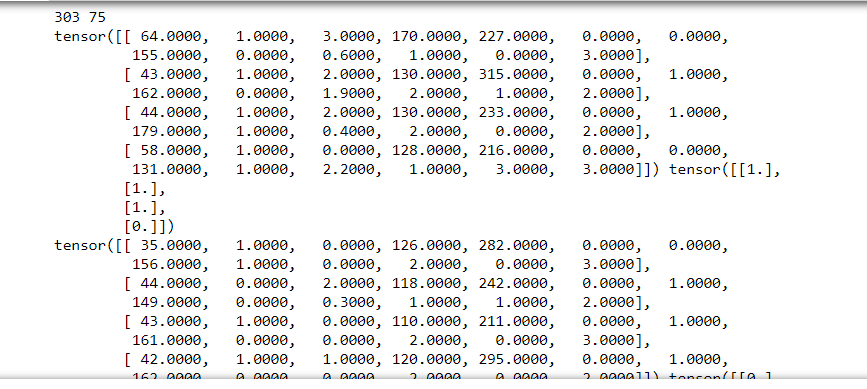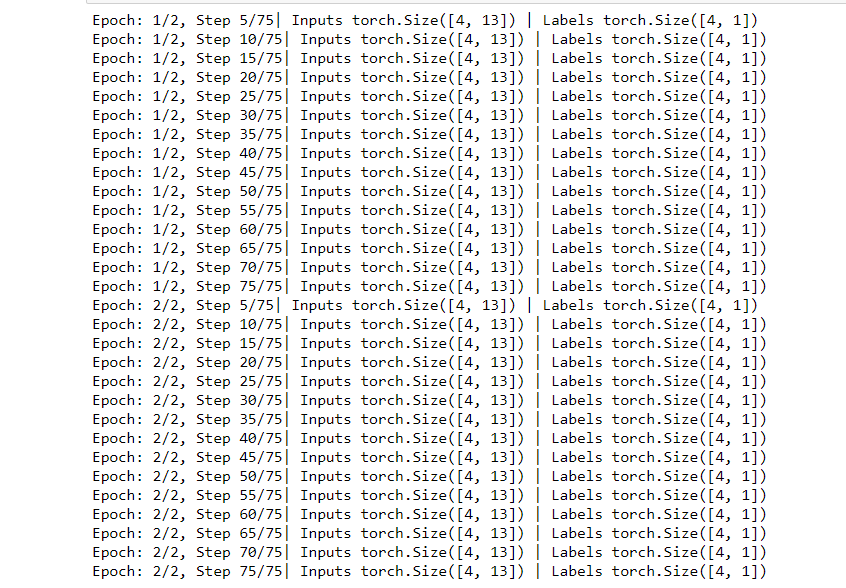# Datasets And Dataloaders in Pytorch

• Last Updated : 18 Jul, 2021

PyTorch is a Python library developed by Facebook to run and train machine learning and deep learning models.  Training a deep learning model requires us to convert the data into the format that can be processed by the model. PyTorch provides the torch.utils.data library to make data loading easy with DataSets and Dataloader class.

Dataset is itself the argument of DataLoader constructor which indicates a dataset object to load from. There are two types of datasets:

• map-style datasets: This data set provides two functions  __getitem__( ), __len__( ) that returns the indices of the sample data referred to and the numbers of samples respectively. In the example, we will use this type of dataset.
• iterable-style datasets: Datasets that can be represented in a set of iterable data samples, for this we use __iter__( )function.

Dataloader on the other hand, not only allows us to iterate through the dataset in batches but also gives us access to inbuilt functions for multiprocessing(allows us to load multiple batches of data in parallel, rather than loading one batch at a time), shuffling, etc.

Syntax:

DataLoader(dataset, batch_size=1, shuffle=False, sampler=None, batch_sampler=None, num_workers=0, collate_fn=None, pin_memory=False, drop_last=False, timeout=0, worker_init_fn=None, *, prefetch_factor=2, persistent_workers=False)

Dataset Used: heart

Let us deal with an example so that the concept becomes clearer.

First import all required libraries and the dataset to work with. Load dataset in torch tensors which are accessed through __getitem__( ) protocol, to get the index of the particular dataset. Then we unpack the data and print corresponding features and labels.

Example:

## Python3

 `# importing libraries``import` `torch``import` `torchvision``from` `torch.utils.data ``import` `Dataset, DataLoader``import` `numpy as np``import` `math`` ` `# class to represent dataset``class` `HeartDataSet():`` ` `    ``def` `__init__(``self``):``       ` `        ``# loading the csv file from the folder path``        ``data1 ``=` `np.loadtxt(``'heart.csv'``, delimiter``=``','``,``                           ``dtype``=``np.float32, skiprows``=``1``)``         ` `        ``# here the 13th column is class label and rest ``        ``# are features``        ``self``.x ``=` `torch.from_numpy(data1[:, :``13``])``        ``self``.y ``=` `torch.from_numpy(data1[:, [``13``]])``        ``self``.n_samples ``=` `data1.shape[``0``] ``     ` `    ``# support indexing such that dataset[i] can ``    ``# be used to get i-th sample``    ``def` `__getitem__(``self``, index):``        ``return` `self``.x[index], ``self``.y[index]``       ` `    ``# we can call len(dataset) to return the size``    ``def` `__len__(``self``):``        ``return` `self``.n_samples`` ` ` ` `dataset ``=` `HeartDataSet()`` ` `# get the first sample and unpack``first_data ``=` `dataset[``0``]``features, labels ``=` `first_data``print``(features, labels)`

Output:

tensor([ 63.0000,   1.0000,   3.0000, 145.0000, 233.0000,   1.0000,   0.0000,

150.0000,   0.0000,   2.3000,   0.0000,   0.0000,   1.0000]) tensor([1.])

The torch dataLoader takes this dataset as input, along with other arguments for batch_size, shuffle, etc, calculate nums_samples per batch, then print out the targets and labels in batches.

Example:

## Python3

 `# Loading whole dataset with DataLoader``# shuffle the data, which is good for training``dataloader ``=` `DataLoader(dataset``=``dataset, batch_size``=``4``, shuffle``=``True``)`` ` `# total samples of data and number of iterations performed``total_samples ``=` `len``(dataset)``n_iterations ``=` `total_samples``/``/``4``print``(total_samples, n_iterations)``for` `i, (targets, labels) ``in` `enumerate``(dataloader):``    ``print``(targets, labels)`

Output:We now train the data by first looping over the epoch and then over samples after that printing out the number of epochs, input tensor and label tensor with each iteration.

Example:

## Python3

 `num_epochs ``=` `2`` ` `for` `epoch ``in` `range``(num_epochs):``    ``for` `i, (inputs, labels) ``in` `enumerate``(dataloader):`` ` `        ``# here: 303 samples, batch_size = 4, n_iters=303/4=75 iterations``        ``# Run our training process``        ``if` `(i``+``1``) ``%` `5` `=``=` `0``:``            ``print``(f'Epoch: {epoch``+``1``}``/``{num_epochs}, Step {i``+``1``}``/``{n_iterations}|\``                ``Inputs {inputs.shape} | Labels {labels.shape}')`

Output:My Personal Notes arrow_drop_up